# Mathematics All india set 1 2010-2011 CBSE Class 10 Question Paper Solution

Mathematics [All india set 1]
Date: March 2011

1

The roots of the equation x2 − 3x − m (m + 3) = 0, where m is a constant, are

A. mm + 3

B. − mm + 3

C. m, − (m + 3)

D. m, − (m + 3)

2

If the common differences of an A.P. is 3, then a20 − a15 is

A. 5

B. 3

C. 15

D. 20

Concept: Sum of First n Terms of an AP
Chapter: [2.02] Arithmetic Progressions
3

In figure 1, O is the centre of a circle, PQ is a chord and PT is the tangent at P.

If ∠POQ = 70°, then ∠TPQ is equal to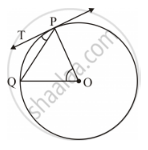A. 55°

B. 70°

C. 45°

D. 35°

Concept: Concept of Circle - Centre, Radius, Diameter, Arc, Sector, Chord, Segment, Semicircle, Circumference, Interior and Exterior.
Chapter: [3.01] Circles [3.01] Circles
4

In Figure 2, AB and AC are tangents to the circle with centre O such that

∠BAC = 40°. Then ∠BOC is equal to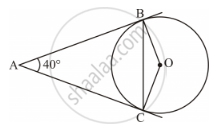A. 40°

B. 50°

C. 140°

D. 150°

Concept: Tangent to a Circle
Chapter: [3.01] Circles
5

The perimeter (in cm) of a square circumscribing a circle of radius a cm, is

A. a

B. a

C. a

D. 16 a

Concept: Concept of Circle - Centre, Radius, Diameter, Arc, Sector, Chord, Segment, Semicircle, Circumference, Interior and Exterior.
Chapter: [3.01] Circles [3.01] Circles
6

The radius (in cm) of the largest right circular cone that can be cut out from a cube of edge 4.2 cm is

A. 4.2

B. 2.1

C. 8.4

D. 1.05

Concept: Heights and Distances
Chapter: [4.01] Heights and Distances
7

A tower stands vertically on the ground. From a point on the ground which is 25 m away from the foot of the tower, the angle of elevation of the top of the tower is found to be 45°. Then the height (in meters) of the tower is

A. 25sqrt2

B. 25sqrt3

C. 25

D. 12.5

Concept: Heights and Distances
Chapter: [4.01] Heights and Distances
8

If P(a/2,4)is the mid-point of the line-segment joining the points A (−6, 5) and B(−2, 3), then the value of a is

A. −8

B. 3

C. −4

D. 4

Concept: Coordinate Geometry Examples and Solutions
Chapter: [6.01] Lines (In Two-dimensions)
9

If A and B are the points (−6, 7) and (−1, −5) respectively, then the distance

2AB is equal to

A. 13

B. 26

C. 169

D. 238

Concept: Distance Formula
Chapter: [6.01] Lines (In Two-dimensions)
10

A card is drawn from a well-shuffled deck of 52 playing cards. The probability that the card will not be an ace is

A. 1/13

B. 1/4

C. 12/13

D.3/4

Concept: Concept of Probability - Random Experiment, Outcome, Equally Likely Outcomes.
Chapter: [5.01] Probability [5.01] Probability
11

Find the value of m so that the quadratic equation mx (x − 7) + 49 = 0 has two equal roots.

Concept: Quadratic Equations Examples and Solutions
12

How many two-digit number are divisible by 6?

Concept: Sum of First n Terms of an AP
Chapter: [2.02] Arithmetic Progressions
13

In Figure 3, a circle touches all the four sides of a quadrilateral ABCD whose sides are AB = 6 cm, BC = 9 cm and CD = 8 cm. Find the length of the side AD.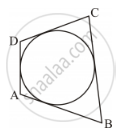Concept: Concept of Circle - Centre, Radius, Diameter, Arc, Sector, Chord, Segment, Semicircle, Circumference, Interior and Exterior.
Chapter: [3.01] Circles [3.01] Circles
14

Draw a line segment AB of length 7 cm. Using ruler and compasses, find a point P on AB such that (AP)/(AB)=3/5.

Concept: Division of a Line Segment
Chapter: [3.03] Constructions
15

Find the perimeter of the shaded region in Figure 4, if ABCD is a square of side 14 cm and APB and CPD are semicircles. Use pi = 22/7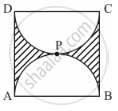Concept: Areas of Combinations of Plane Figures
Chapter: [7.01] Areas Related to Circles
16 | attempt any one of the following
16.1

Two cubes each of volume 27 cm3 are joined end to end to form a solid. Find the surface area of the resulting cuboid.

Concept: Surface Area of a Combination of Solids
Chapter: [7.02] Surface Areas and Volumes
16.2

A cone of height 20 cm and radius of base 5 cm is made up of modeling clay. A child reshapes it in the form of a sphere. Find the diameter of the sphere.

Concept: Frustum of a Cone
Chapter: [7.02] Surface Areas and Volumes
17

Find the value of y for which the distance between the points A (3, −1) and B (11, y) is 10 units.

Concept: Distance Formula
Chapter: [6.01] Lines (In Two-dimensions)
18

A ticket is drawn at random from a bag containing tickets numbered from 1 to 40. Find the probability that the selected ticket has a number which is a multiple of 5.

Concept: Simple Problems on Single Events
Chapter: [5.01] Probability
19

Find the roots of the following quadratic equation:

x^2-3sqrt5x+10=0

20

Find the A.P. whose fourth term is 9 and the sum of its sixth term and thirteenth term is 40.

Concept: Sum of First n Terms of an AP
Chapter: [2.02] Arithmetic Progressions
21

In Figure 5, a triangle PQR is drawn to circumscribe a circle of radius 6 cm such that the segments QT and TR into which QR is divided by the point of contact T, are of lengths 12 cm and 9 cm respectively. If the area of ΔPQR = 189 cm2, then find the lengths of sides PQ and PR.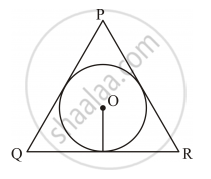Concept: Tangent to a Circle
Chapter: [3.01] Circles
22 | attempt any one of the following
22.1

Draw a pair of tangents to a circle of radius 3 cm, which are inclined to each other at an angle of 60°.

Concept: Construction of Tangents to a Circle
Chapter: [3.03] Constructions
22.2

Draw a right triangle in which the sides (other than the hypotenuse) are of lengths 4 cm and 3 cm. Now construct another triangle whose sides are 3/5 times the corresponding sides of the given triangle.

Concept: Division of a Line Segment
Chapter: [3.03] Constructions
23

A chord of a circle of radius 14 cm subtends an angle of 120° at the centre. Find the area of the corresponding minor segment of the circle. [User pi22/7 and sqrt3=1.73]

Concept: Concept of Circle - Centre, Radius, Diameter, Arc, Sector, Chord, Segment, Semicircle, Circumference, Interior and Exterior.
Chapter: [3.01] Circles [3.01] Circles
24

An open metal bucket is in the shape of a frustum of a cone of height 21 cm with radii of its lower and upper ends as 10 cm and 20 cm respectively. Find the cost of milk which can completely fill the bucket at Rs. 30 per litre. [User pi22/7]

Concept: Frustum of a Cone
Chapter: [7.02] Surface Areas and Volumes
25

Point P(x, 4) lies on the line segment joining the points A(−5, 8) and B(4, −10). Find the ratio in which point P divides the line segment AB. Also find the value of x.

Concept: Concepts of Coordinate Geometry
Chapter: [6.01] Lines (In Two-dimensions)
26 | attempt any one of the following
26.1

Find the area of the quadrilateral ABCD, whose vertices are A(−3, −1), B (−2, −4), C(4, − 1) and D (3, 4).

Concept: Coordinate Geometry Examples and Solutions
Chapter: [6.01] Lines (In Two-dimensions)
26.2

Find the area of triangle formed by joining the mid-points of the sides of the triangle whose vertices are A(2, 1), B(4, 3) and C(2, 5).

Concept: Areas of Combinations of Plane Figures
Chapter: [7.01] Areas Related to Circles
27

From the top of a vertical tower, the angles depression of two cars in the same straight line with the base of the tower, at an instant are found to be 45°  and 60° . If the cars are 100 m apart and are on the same side of the tower, find the height of the tower.

Concept: Heights and Distances
Chapter: [4.01] Heights and Distances
28 | attempt any one of the following
28.1

Two dice are rolled once. Find the probability of getting such numbers on the two dice, whose product is 12.

Concept: Concept of Probability - Random Experiment, Outcome, Equally Likely Outcomes.
Chapter: [5.01] Probability [5.01] Probability
28.2

A box contains 80 discs which are numbered from 1 to 80. If one disc is drawn at random from the box, find the probability that it bears a perfect square number.

Concept: Concept of Probability - Random Experiment, Outcome, Equally Likely Outcomes.
Chapter: [5.01] Probability [5.01] Probability
29

Prove that the tangent at any point of a circle is perpendicular to the radius through the point of contact.

Concept: Tangent to a Circle
Chapter: [3.01] Circles
30 | attempt any one of the following
30.1

The first and the last terms of an A.P. are 8 and 350 respectively. If its common difference is 9, how many terms are there and what is their sum?

Concept: Sum of First n Terms of an AP
Chapter: [2.02] Arithmetic Progressions
30.2

How many multiples of 4 lie between 10 and 250?

Concept: nth Term of an AP
Chapter: [2.02] Arithmetic Progressions
31 | attempt any one of the following
31.1

A train travels 180 km at a uniform speed. If the speed had been 9 km/hr more, it would have taken 1 hour less for the same journey. Find the speed of the train.

Concept: Situational Problems Based on Quadratic Equations Related to Day to Day Activities to Be Incorporated
31.2

Find the roots of the equation  .1/(2x-3)+1/(x+5)=1,x≠3/2,5

Concept: Nature of Roots
32

In following figure, three circles each of radius 3.5 cm are drawn in such a way that each of them touches the other two. Find the area enclosed between these three circles (shaded region). ["Use" pi=22/7]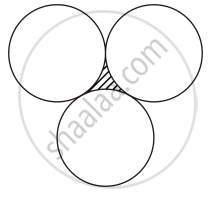Concept: Concept of Circle - Centre, Radius, Diameter, Arc, Sector, Chord, Segment, Semicircle, Circumference, Interior and Exterior.
Chapter: [3.01] Circles [3.01] Circles
33

Water is flowing at the rate of 15 km/hour through a pipe of diameter 14 cm into a cuboidal pond which is 50 m long and 44 m wide. In what time will the level of water in the pond rise by 21 cm?

Concept: Concept of Surface Area, Volume, and Capacity
Chapter: [7.02] Surface Areas and Volumes [7.02] Surface Areas and Volumes
34

The angle of elevation of the top of a vertical tower from a point on the ground is 60° . From another point 10 m vertically above the first, its angle of elevation is 30° .Find the height of the tower.

Concept: Heights and Distances
Chapter: [4.01] Heights and Distances

#### Request Question Paper

If you dont find a question paper, kindly write to us

View All Requests

#### Submit Question Paper

Help us maintain new question papers on Shaalaa.com, so we can continue to help students

only jpg, png and pdf files

## CBSE previous year question papers Class 10 Mathematics with solutions 2010 - 2011

CBSE Class 10 Maths question paper solution is key to score more marks in final exams. Students who have used our past year paper solution have significantly improved in speed and boosted their confidence to solve any question in the examination. Our CBSE Class 10 Maths question paper 2011 serve as a catalyst to prepare for your Mathematics board examination.
Previous year Question paper for CBSE Class 10 Maths-2011 is solved by experts. Solved question papers gives you the chance to check yourself after your mock test.
By referring the question paper Solutions for Mathematics, you can scale your preparation level and work on your weak areas. It will also help the candidates in developing the time-management skills. Practice makes perfect, and there is no better way to practice than to attempt previous year question paper solutions of CBSE Class 10.

How CBSE Class 10 Question Paper solutions Help Students ?
• Question paper solutions for Mathematics will helps students to prepare for exam.
• Question paper with answer will boost students confidence in exam time and also give you an idea About the important questions and topics to be prepared for the board exam.
• For finding solution of question papers no need to refer so multiple sources like textbook or guides.